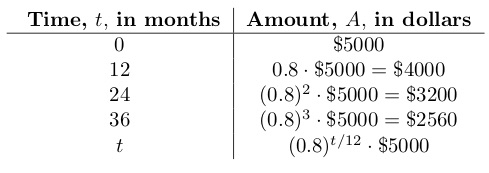# Exponential Decay

## Problem

Jeff invests \$5000 in a stock. He thinks his investment will grow, but he knows there is a risk that it will lose value. He estimates that at worst the value will decrease by 20% every 12 months.

Write an expression to represent Jeff’s worst case estimate of the stock’s value t months after he makes the investment.## Solution

A problem like this, in which a quantity decreases by the same fraction of itself every week or month or year or 12 years, is called exponential decay. (A similar problem, in which a quantity increases by the same fraction of itself in a given time period, is called exponential growth.)

If Jeff’s worst nightmare plays out, the stock will lose 20% of its value in 12 months. That means that after 12 months, the stock will be worth 1 – 0.2 = 0.8 of its original value. Its value will get multiplied by 0.8 for every 12 months that pass.

Every 12 months the value will be decreased to 0.8 of its earlier value — that is, multiplied by 0.8 of its value — so that after n periods of 12 months each, the value, A, will be the original value, , multiplied by . In exponential decay, the number that gets taken to a power — 0.8 in this case — is called the decay factor.

But the question asks for the value t months, not n periods of 12 months, after the investment is made. Since n is a period of 12 months and t represents 1 month,  Then .